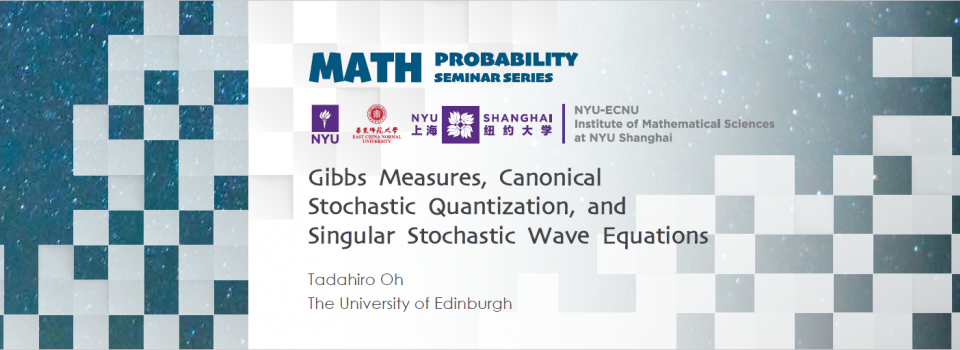# Gibbs Measures, Canonical Stochastic Quantization, and Singular Stochastic Wave EquationsTopic:
Gibbs Measures, Canonical Stochastic Quantization, and Singular Stochastic Wave Equations
Date & Time:
Thursday, November 17, 2022 - 17:30 to 18:30
Speaker:
Tadahiro Oh, The University of Edinburgh
Location:

- RSVP Here -

Abstract:

In this talk, I will discuss the (non-)construction of the focusing Gibbs measures and the associated dynamical problems. This study was initiated by Lebowitz, Rose, and Speer (1988) and continued by Bourgain (1994), Brydges-Slade (1996), and Carlen-Fröhlich-Lebowitz (2016). In the one-dimensional setting, we consider the mass-critical case, where a critical mass threshold is given by the mass of the ground state on the real line. In this case, I will show that the Gibbs measure is indeed normalizable at the optimal mass threshold, thus answering an open question posed by Lebowitz, Rose, and Speer (1988).

In the three dimensional-setting, I will first discuss the construction of the \$\Phi^3_3\$-measure with a cubic interaction potential. This problem turns out to be critical, exhibiting a phase transition: normalizability in the weakly nonlinear regime and non-normalizability in the strongly nonlinear regime. Then, I will discuss the dynamical problem for the canonical stochastic quantization of the \$\Phi^3_3\$-measure, namely, the three-dimensional stochastic damped nonlinear wave equation with a quadratic nonlinearity forced by an additive space-time white noise (= the hyperbolic \$\Phi^3_3\$-model). As for the local theory, I will describe the paracontrolled approach to study stochastic nonlinear wave equations, introduced in my work with Gubinelli and Koch (2018). In the globalization part, I introduce a new, conceptually simple and straightforward approach, where we directly work with the (truncated) Gibbs measure, using the variational formula and ideas from theory of optimal transport.

If time permits, I will describe other related problems/results.

The first part of the talk is based on a joint work with Philippe Sosoe (Cornell) and Leonardo Tolomeo (Bonn/Edinburgh), while the second part is based on a joint work with Mamoru Okamoto (Osaka) and Leonardo Tolomeo (Bonn/Edinburgh).

Biography:

Professor Tadahiro Oh is a Professor at the University of Edinburgh, UK. Before joining the University of Edinburgh, he held academic positions as an Assistant Professor at Princeton University, USA, and a postdoctoral researcher at the University of Toronto, Canada. He works in the field lying at the intersection of dispersive PDEs and stochastic analysis, and is known for his work on singular stochastic dispersive PDEs.  He was awarded an ERC Starting Grant (2014), an ERC Consolidator Grant (2019), and the Whitehead Prize (2022).

Seminar by the NYU-ECNU Institute of Mathematical Sciences at NYU Shanghai